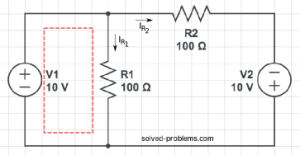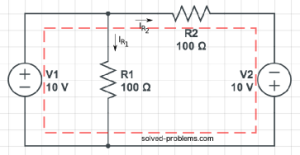Find resistor currents using KVL.Solution:

$R_1$ and $V_1$ are parallel. So the voltage across $R_1$ is equal to $V_1$. This can be also calculated using KVL in the left hand side loop:$-V_1+V_{R_1}=0 \rightarrow V_{R_1}=V_1=10V$.
Now, use Ohm's law to find $I_{R_1}$:

$V_{R_1}=R_1 \times I_{R_1} \rightarrow I_{R_1}=\frac{V_{R_1}}{R_1}=0.1 A$.
To find $I_{R_2}$, write KVL around the outer loop:$-V_1+V_{R_2}-V_2=0 \rightarrow V_{R_2}=V_1+V_2=20V$.
Again, use Ohm's law to determine $I_{R_2}$:

$V_{R_2}=R_2 \times I_{R_2} \rightarrow I_{R_2}=\frac{V_{R_2}}{R_2}=0.2 A$.

Now, tell me what is the current passing through $V_1$?

Hi! Yaz is here. I am passionate about learning and teaching. I try to explain every detail simultaneously with examples to ensure that students will remember them later too.

## Join the Conversation

1.2.3.4.5.6.1. 0.3

1. good job!
From KCL:
$I_{V_1}=I_{R_1}+I_{R_2}=0.3 A$

2.yongule says:

good work i got aleast some concepts

1. good, very good. let me know if you need any help.

1.shahidul islam says:

My Circuit

1.$10 \Omega$ and $3 \Omega$ are both short circuited. If you check the circuit you can easily see that both terminals are connected to each other for both.
So, you have a circuit with a $10 V$ source and $2 \Omega$ and Ohm's law says:
$I=\frac{V}{R}=5 A$
Let me know if you need more help.

1.shahidul islam says:

Thank you very much, sir.

Surprisingly, in every guidebook I found the answer 7.5 Amp where there was no explanation behind the answer. But now I know the explanation and also know that correct answer is 5 Amp.

I will post here if I need further help. Thanks again for your help.

2. You are welcome.

2.prasad says:

eliminate 10ohm resister becuase current will flow through low resistance patch then it is a series resistance circuit easy you can calculate otherwise let me know

3.Thomas says:

What happens if you reverse the polarity of V2?

1. good question.
Actually, it will be quite interesting. The voltage across $R_1$ is $10 V$ and therefore, the voltage drop on $R_2$ will be zero. You can also verify this by KVL around the outer loop:
$-10 V +V_{R_2}+10 V=0 \rightarrow V_{R_2}=0$
And using the Ohm's law, we conclude that no current passes through $R_2$. The current of $R_1$ is the same as before because its voltage is not changed.

4.Min Dhami says:

please give me solve two voltage source are given